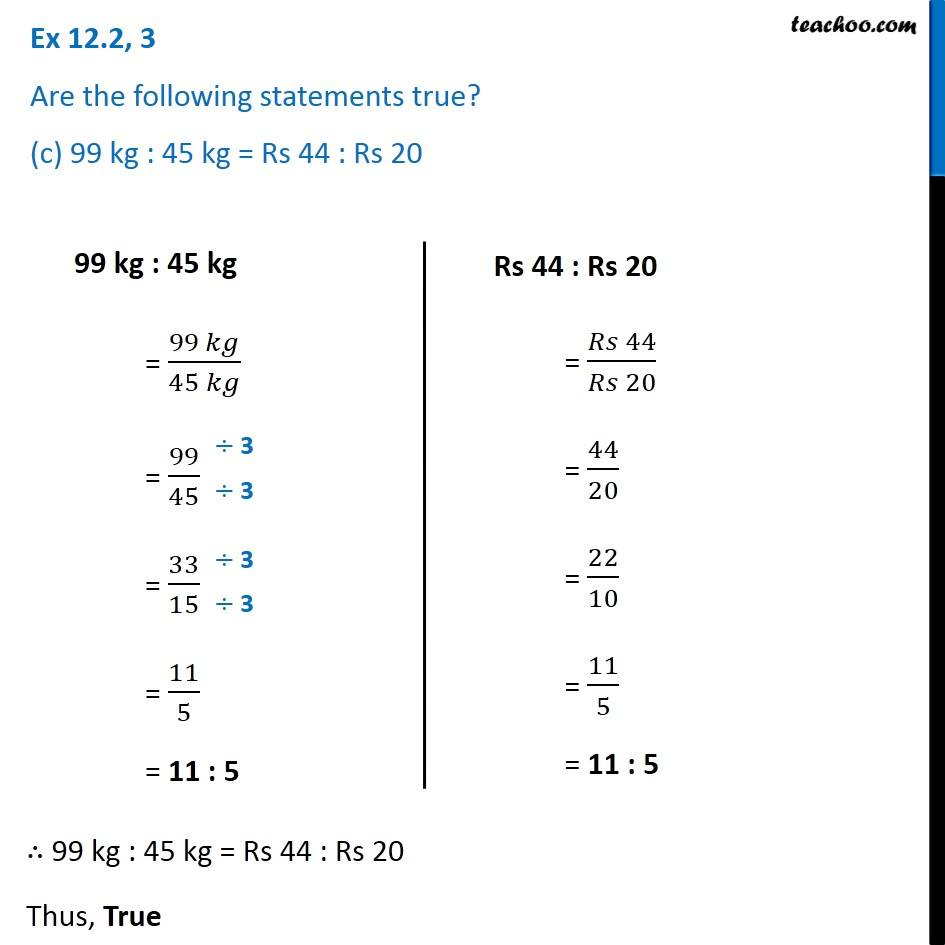Ex 12.2

Chapter 12 Class 6 Ratio and Proportion
Serial order wiseLearn in your speed, with individual attention - Teachoo Maths 1-on-1 Class

### Transcript

Ex 12.2, 3 Are the following statements true? (c) 99 kg : 45 kg = Rs 44 : Rs 2099 kg : 45 kg = (99 𝑘𝑔)/(45 𝑘𝑔) = 99/45 = 33/15 = 11/5 = 11 : 5 Rs 44 : Rs 20 = (𝑅𝑠 44)/(𝑅𝑠 20) = 44/20 = 22/10 = 11/5 = 11 : 5 ∴ 99 kg : 45 kg = Rs 44 : Rs 20 Thus, True